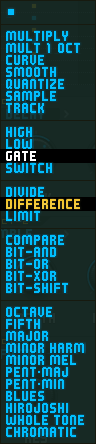# ArcSyn User Manual: Mod Modifiers• The Modulation Matrix Modifiers allow modulation sources A and B to be combined together and processed in different ways.
• These Modifiers are an 'advanced' or 'expert' feature which you don't need to use to get interesting sounds out of ArcSyn.
• For in-tune pitch modulation, set AMOUNT to 100% (or -100% for in-tune but upside-down modulation).

### OFF

A and B added together. This allows either or both sources to be used without modification.

### MULTIPLY

A multiplied by B. Useful for such basic routings as mod-wheel control of oscillator pitch modulation.

### MULT 1 OCT

A multipled by B then scaled so that the maximum pitch modulation amount is 1 octave when AMOUNT is set to 100%.

### CURVE

A 'curved' (or 'warped') by B. If B is zero, A is unmodified. If B is positive, A is bent into a convex curve, if B is negative, A is bent into a concave curve.

### SMOOTH

A smoothed (LP filtered) by B. If B is zero or negative, A is unmodified. If B is positive, A is smoothed. The higher B goes, the more A is smoothed.

### QUANTIZE

A quantised (made into steps) by B. If B is zero or negative, A is unmodified. If B is positive, A is quantised into steps which are the same size as B, so if B is 0.1 and A is an increasing ramp, the output of this modifier will be steps of zero, 0.1, 0.2, 0.3 and so on. TIP: if B is a level which is an exact musical amount like a minor third (from an LFO DC waveform with a level set to 3), A will be quantised into equal musical steps.

### SAMPLE

A sampled (like a sample-and-hold) by B. If B increases from zero to more than zero, A is sampled and the output of this modifier is then held at the same level until the next time B increases from zero to more than zero. If B never rises above zero, the output of this modifier will remain at zero.

### TRACK

A tracked by B. If B is more than zero, A is output. If B is less than zero, A is sampled and held.

### HIGH

Outputs the highest input. If A is 0.5 and B is 0.1, the output will be 0.5.

### LOW

Outputs the lowest input. If A is 0.5 and B is 0.1, the output will be 0.1.

### GATE

A if more than B, otherwise zero.

### SWITCH

A if B more than zero, otherwise zero. B is used to switch A on and off.

### DIVIDE

A divided by B with the output limited to +/-1 to avoid divide-by-zero errors.

### DIFFERENCE

A minus B but converted so it's always positive. If A is 0.5 and B is 0.1, the output will be 0.4. If A is 0.1 and B is 0.5, the output will still be 0.4.

### LIMIT

A limited by the level by B. A is limited equally whether it's positive or negative, so if B is -0.5 and A is 1, A will be limited to 0.5.

### COMPARE

If A is higher than B, the output is 1, if B is higher than A, the output is 0.

### BIT-SHIFT

These 4 modifiers work by converting A and B sources to 8 bit binary numbers, with a range from zero to 255. The numbers are then combined using different binary maths operations.

### CHROMATIC

The last 11 modifiers add A and B together then quantise them to musical scales. As usual, you'll need to set the modulation AMOUNT slider to 100% for the modulation to be in tune. (Assuming that's what you want! Try choosing the CHROMATIC scale then setting the modulation AMOUNT slider to 50%—you'll get quarter-tone steps.)

Go to top